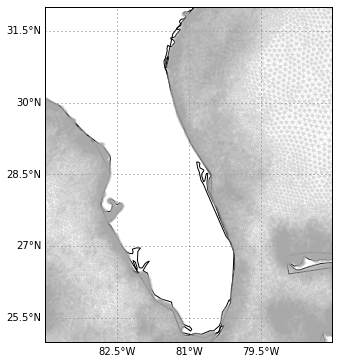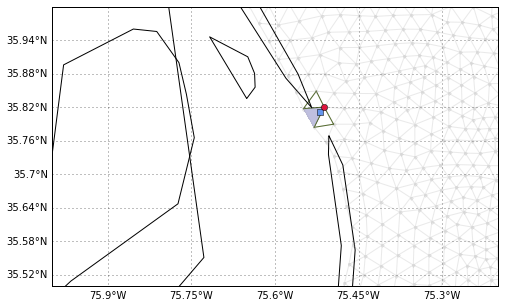# SciPy 2015 sprint: pyugrid

Last week, at the SciPy conference, I had chance to sprint on the pyugrid. The pyugrid module aims to be a Python API to utilize data written using the netCDF unstructured grid conventions. Right now it support only triangular meshes, but the conventions cover any type of unstructured grids.

Let's explore this module in this post. First: how to read an unstructured?

(And once hexagonal meshes are implemented I will be able to re-create Borges' Library of Babel and solved the crime using network theory and the connectivity array ;-)

In :
import pyugrid

print(pyugrid.__version__)

url = ('http://comt.sura.org/thredds/dodsC/data/comt_1_archive/'
'inundation_tropical/VIMS_SELFE/Hurricane_Ike_3D_final_run_with_waves')

ugrid = pyugrid.UGrid.from_ncfile(url)

0.1.5



How about we check this dataset for consistency?

In :
ugrid.check_consistent()

---------------------------------------------------------------------------
NotImplementedError                       Traceback (most recent call last)
----> 1 ugrid.check_consistent()

/home/filipe/.virtualenvs/blog/lib/python2.7/site-packages/pyugrid/ugrid.py in check_consistent(self)
161         existing nodes, etc.
162         """
--> 163         raise NotImplementedError
164
165     @property

NotImplementedError: 

Oh, oh... I guess we should have worked harder during the sprint =)

OK, since this is a triangular mesh lets read read the topology into something matplotlib users know (x, y, triangles).

In :
lon = ugrid.nodes[:, 0]
lat = ugrid.nodes[:, 1]
triangles = ugrid.faces[:]


The dataset has nodes (or vertexes) representing a point in a 2D space, the faces (or polygons) correspond to a plane enclosed by a set of edges. Note that the dataset does not contain the edges! We need to compute those:

In :
%time ugrid.build_edges()

CPU times: user 3.9 s, sys: 64 ms, total: 3.96 s
Wall time: 3.95 s



~4 seconds is kind of slow. Since this grid has a 2D triangular mesh topology one might ask: how does the calculated edges compare with matplotlib triangulation? Let's check it out!

In :
import matplotlib.tri as tri

%time triang = tri.Triangulation(lon, lat, triangles=triangles)

CPU times: user 8 ms, sys: 1 ms, total: 9 ms
Wall time: 9.01 ms



matplotlib create the triang object faster than pyugrid. Let's take a closer look to see if the computed edges are similar.

In :
ugrid.edges.shape == triang.edges.shape

Out:
True

In :
ugrid.edges == triang.edges

Out:
array([[False, False],
[False, False],
[False, False],
...,
[False, False],
[False, False],
[False, False]], dtype=bool)


OK. The edge matrix is different :-(, that is probably just the ordering of the elements though.

Let's take a quick look at the mesh.

In :
import cartopy.crs as ccrs
import matplotlib.pyplot as plt
from cartopy.mpl.gridliner import LONGITUDE_FORMATTER, LATITUDE_FORMATTER

def make_map(projection=ccrs.PlateCarree()):
fig, ax = plt.subplots(figsize=(8, 6),
subplot_kw=dict(projection=projection))
ax.coastlines(resolution='50m')
gl = ax.gridlines(draw_labels=True)
gl.xlabels_top = gl.ylabels_right = False
gl.xformatter = LONGITUDE_FORMATTER
gl.yformatter = LATITUDE_FORMATTER
return fig, ax

In :
fig, ax = make_map()

kw = dict(marker='.', linestyle='-', alpha=0.25, color='darkgray')
ax.triplot(triang, **kw)  # or lon, lat, triangules
ax.coastlines()
ax.set_extent([-84, -78, 25, 32])Let's try to find the point nearest to one of the SECOORA buoys in the dataset.

In :
duck = [-75.51997, 35.8112]

In :
%%time
duck_face = ugrid.locate_face_simple(duck)

print(duck_face)

848669
CPU times: user 40.6 s, sys: 0 ns, total: 40.6 s
Wall time: 40.6 s



Ouch! That took way too long. Let's see if matplotlib is faster.

In :
%%time
f = triang.get_trifinder()

print(f(duck, duck))

848669
CPU times: user 9.69 s, sys: 226 ms, total: 9.92 s
Wall time: 9.91 s



Yep! matplotlib is not only faster but subsequent calls will instantaneous since the TriFinder object is cached.

In :
%time duck_node = ugrid.locate_nodes(duck)

CPU times: user 84 ms, sys: 5 ms, total: 89 ms
Wall time: 88.9 ms



That was much faster! Locating nodes uses a cached KDTree for optimization.

Both pyugrid and matplotlib can return the face-face connectivity array (the neighbors of each triangle). The connectivity array can be extremely useful when searching for the shortest path between to grid points. Again, lets check if they are similar.

In :
ugrid.build_face_face_connectivity()

(ugrid.face_face_connectivity == triang.neighbors).all()

Out:
True

In :
neighbors = triang.neighbors[duck_face]
neighbors

Out:
array([848668,     -1, 848670], dtype=int32)


This triangle has only two neighbors. Let's check if this is correct by plotting everything we found before ending this post.

In :
import numpy as np

def plt_triangle(triang, face, ax=None, **kw):
if not ax:
fig, ax = plt.subplots()
ax.triplot(triang.x[triang.triangles[face]],
triang.y[triang.triangles[face]],
triangles=triang.triangles[face], **kw)

In :
fig, ax = make_map()

ax.triplot(triang, **kw)
ax.plot(duck, duck, color='cornflowerblue',
marker='s', zorder=2)
ax.plot(lon[duck_node], lat[duck_node], color='crimson',
marker='o', zorder=2)

# Station.
ax.tripcolor(triang.x[triang.triangles[duck_face]], triang.y[triang.triangles[duck_face]],
triang.triangles[duck_face], facecolors=np.array(), alpha=0.25, zorder=1)

# Neighbors.
plt_triangle(triang, neighbors, ax=ax, color='darkolivegreen', zorder=1)
plt_triangle(triang, neighbors[-1], ax=ax, color='darkolivegreen', zorder=1)

ax.coastlines()
ax.set_extent([-76, -75.2, 35.5, 36])

/home/filipe/.virtualenvs/blog/lib/python2.7/site-packages/matplotlib/tri/triangulation.py:110: FutureWarning: comparison to None will result in an elementwise object comparison in the future.
self._neighbors)Everything looks about right. We found the node (red circle) and the triangle (blue) nearest our buoy (blue square). We also found the neighboring triangles (green).

The best feature of pyugrid is that the library interprets the UGrid conventions for you when reading/saving unstructured grids (see this example). However, when it comes to manipulating the grid, pyugrid is lagging behind matplotlib.

BTW: matplotlib's TriAnalyzer packs some neat methods to analyze the triangular mesh.

In :
HTML(html)

Out:

This post was written as an IPython notebook. It is available for download or as a static html.